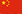Welcome To Our Company中文
You are here: » » » The Combined Gas Law in Chemistry

# The Combined Gas Law in Chemistry

Views: 6     Author: Anne Marie Helmenstine, Ph.D.     Publish Time: 2023-02-01      Origin: ThoughtCo.

The Combined Gas Law in Chemistry

By Anne Marie Helmenstine, Ph.D.

Updated on November 19, 2022The combined gas law combines the three gas laws: Boyle's Law, Charles' Law, and Gay-Lussac's Law. It states that the ratio of the product of pressure and volume and the absolute temperature of a gas is equal to a constant. When Avogadro's law is added to the combined gas law, the ideal gas law results. Unlike the named gas laws, the combined gas law doesn't have an official discoverer. It is simply a combination of the other gas laws that works when everything except temperature, pressure, and volume are held constant.Combined Gas Law Formulas

There are a couple of common equations for writing the combined gas law. The classic law relates Boyle's law and Charles' law to state:

PV/T = k

where P = pressure, V = volume, T = absolute temperature (Kelvin), and k = constant.

The constant k is a true constant if the number of moles of the gas doesn't change. Otherwise, it varies.

Another common formula for the combined gas law relates "before and after" conditions of a gas:

P1V1 / T1 = P2V2 / T2

Example

Find the volume of a gas at STP when 2.00 liters is collected at 745.0 mm Hg and 25.0 degrees Celsius.

To solve the problem, you first need to identify which formula to use. In this case, the question asks about conditions at STP, so you know you're dealing with a "before and after" problem. Next, you need to understand STP. If you haven't memorized this already (and you probably should, since it appears a lot), STP refers to "standard temperature and pressure," which is 273 Kelvin and 760.0 mm Hg.

Because the law works using absolute temperature, you need to convert 25.0 degrees Celsius to the Kelvin scale. This gives you 298 Kelvin.

At this point, you can plug the values into the formula and solve for the unknown. A common mistake some people make when they're new to this kind of problem is confusing which numbers go together. It's good practice to identify the variables. In this problem they are:

P1 = 745.0 mm Hg

V1 = 2.00 L

T1 = 298 K

P2 = 760.0 mm Hg

V2 = x (the unknown you're solving for)

T2 = 273 K

Next, take the formula and set it up to solve for the unknown "x," which in this problem is V2:

P1V1 / T1 = P2V2 / T2

Cross-multiply to clear the fractions:

P1V1T2 = P2V2T1

Divide to isolate V2:

V2 = (P1V1T2) / (P2T1)

Plug in the numbers and solve for V2:

V2 = (745.0 mm Hg &middot; 2.00 L &middot; 273 K) / (760 mm Hg &middot; 298 K)

V2 = 1.796 L

Report the result using the correct number of significant figures:

V2 = 1.80 L

Applications

The combined gas law has practical applications when dealing with gases at ordinary temperatures and pressures. Like other gas laws based on ideal behavior, it becomes less accurate at high temperatures and pressures. The law is used in thermodynamics and fluid mechanics. For example, it can be used to calculate pressure, volume, or temperature for the gas in clouds to forecast weather.

## GET IN TOUCH

  Yonghua
  yonghua@yonghuachem.com
+86-13776212318
+86-86-512-52890563
Room B-1308, International Trade Center, No. 12 Huanghe Road, Changshu City, Jiangsu Province

## Be the first to know about our lastest products.

Copyright 2020 Yonghua Chemical Co., Ltd.